Courses

# Fluid Mechanics - 3

## 20 Questions MCQ Test Mock Test Series for SSC JE Mechanical Engineering | Fluid Mechanics - 3

Description
This mock test of Fluid Mechanics - 3 for SSC helps you for every SSC entrance exam. This contains 20 Multiple Choice Questions for SSC Fluid Mechanics - 3 (mcq) to study with solutions a complete question bank. The solved questions answers in this Fluid Mechanics - 3 quiz give you a good mix of easy questions and tough questions. SSC students definitely take this Fluid Mechanics - 3 exercise for a better result in the exam. You can find other Fluid Mechanics - 3 extra questions, long questions & short questions for SSC on EduRev as well by searching above.
QUESTION: 1

### The major loss of hydraulic energy in pipe flow occurs in long pipe due to:-

Solution:

There are generally two types of losses occur in a pipe flow problem:

a) Major loss: Major head loss occurs due to friction, which is given by: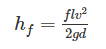Where f = friction factor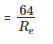1. Sudden enlargement

2. Sudden contraction

3. Due to pipe bends

4. head loss at the entrance and exit of pipe etc.

QUESTION: 2

### An orifice is said to be large, if

Solution:

An orifice is a small aperture through which the fluid passes. The thickness of an orifice in the direction of flow is very small in comparison to its other dimensions. An orifice is said to be large, if the available head of liquid is less than 5 times the height of orifice.

QUESTION: 3

### Reynolds number is the ratio of the inertia force to the:

Solution: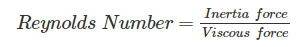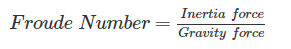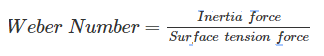QUESTION: 4

For a vacuum pressure of 4.5 m of water, the equivalent absolute pressure is:

Solution:

Absolute pressure = gauge pressure + local atmospheric pressure

-ve gauge pressure = vacuum pressure = 4.5 m of water

Local atmospheric pressure of water = 10.3 m of water

Equivalent absolute pressure = -4.5 + 10.33 = 5.83 m of water

QUESTION: 5

Uniform flow occurs when:

Solution:

The flow is defined as uniform flow when in the flow field the velocity and other hydrodynamic parameters do not change from point to point at any instant of time. For a uniform flow, the velocity is a function of time only. When the velocity and other hydrodynamic parameters changes from one point to another the flow is defined as non-uniform.

QUESTION: 6

The discharge over the rectangular weir is equal to:

Solution:

Discharge over a rectangular weir: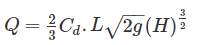Where H: - still water head

Flow over a triangular weir (V-weir):θ: Included angel of Notch.

Flow over a trapezoidal weir (or) Notch-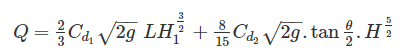Where,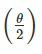: weir angle of inclination with the vertical.

Cd1Cd1 = Coefficient of discharge for rectangular portion.

Cd2Cd2 = Coefficient of discharge for the triangular portion.

QUESTION: 7

Discharge Q in a triangular weir varies as:-

Solution:

Discharge through a triangular notch/weir is given by: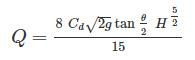Where,

H = height of liquid above apex of the notch

θ = Angle of notch

Cd = Coefficient of discharge

QUESTION: 8

Which manometer is called as simple monometer?

Solution:

A simple manometer consists of a glass tube having one of its ends connected to a point where pressure is to be measured and another end remains open to atmosphere. Common types of simple manometers are:

• Piezometer
• U-tube Manometer
• Single Column Manometer

Differential Manometers measure difference of pressure between two points in a fluid system and cannot measure the actual pressures at any point in the system. It consists of a U-tube, containing a heavy liquid, whose two ends are connected to the points whose difference of pressure is to be measured.

• Upright U-Tube manometer
• Inverted U-Tube manometer
• Inclined Differential manometer
• Micro manometer
QUESTION: 9

In order, to avoid separation in Venturi meter the angle of divergence is kept

Solution: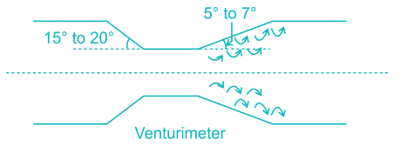In the diverging portion there is a decrease in velocity & subsequent increase in pressure. If divergence angle is very large, then back pressure will increase by great extent & eddies formation will take place resulting in flow separation. Thus, to avoid flow separation the divergence angle must not exceed more than 7° and range should be around 5 - 7°.

QUESTION: 10

The head produced by a liquid of relative density 1.62 is equal to the pressure of 250 kPa. What is the head (m) produced by the liquid?

Solution:

Solution:

Relative density of liquid = 1.62

Pressure = 250 kpa

P = ρgh

250 × 103 = 1.62 × 1000 × 9.81 × h

h = 15.73 m

QUESTION: 11

Time required to empty uniform rectangular tank is proportional to its

Solution: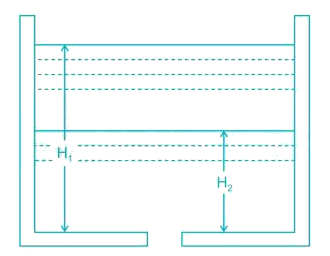A = Surface area of the tank

H1 = initial height of the liquid

H2 = Final height of the liquid

a = Area of the orifice

Now the total time T required to bring the liquid level from H1 to H2 may be found out by integrating the equation between the limits H1 to H2 i.e.,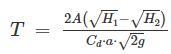If the tank is to be completely emptied, the putting H2 = 0 in this equation, we get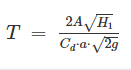QUESTION: 12

Quick sand comes under:

Solution:

Newtonian Fluids: Air, water, mercury, glycerine, kerosene and other engineering fluids under normal circumstances.

Pseudoplastic: Fine particle suspension, gelatine, blood, milk, paper pulp, polymeric solutions such as rubbers, paints.

Dilatant fluids: Ultra fine irregular particle suspension, sugar in water, aqueous suspension of rice starch, quicksand, butter printing ink.

Ideal plastics or Bingham fluids: Sewage sludge, drilling muds.

Viscoelastic fluids: Liquids solid combination in pipe flow, bitumen, tar, asphalt, polymerized fluids with drag reduction features.

Thixotropic: Printer’s ink, crude oil, lipstick, certain paints and enamels.

Rheopectic fluids: Very rare liquid - solid suspensions, gypsum suspension in water and bentonite solutions.

QUESTION: 13

The specific gravity of liquids is usually measured by means of a:

Solution:

A hydrometer or areometer is an instrument that measures the specific gravity (relative density) of liquids-the ratio of the density of the liquid to the density of water.

A hygrometer, also known as a psychrometer, is a device that is used to measure the humidity in the air.

A thermometer is a device that measures temperature or a temperature gradient.

A piezometer is a device used to measure liquid pressure in a system by measuring the height to which a column of the liquid rises against gravity.

QUESTION: 14

The correct statement about ideal fluid is:

Solution:

A fluid is said to be ideal if it assumed to be both incompressible and non-viscous, its bulk modulus is infinite. An ideal fluid is a fluid that has several properties including the fact that it is:

Incompressible - the density is constant

Non-viscous - (Inviscid) fluid has no internal friction.

QUESTION: 15

The type of fluid in which flow and fluid properties does not change with time at any given location, is known as______.

Solution:

Non - uniform Flow: When the fluid properties do not change with respect to space, the type of flow is known as Non - uniform flow.

Steady Flow: When the fluid properties do not change with respect to time, then the flow is known as steady flow.

Unsteady flow: When the fluid properties changes with respect to time, then the flow is known as steady flow.

Rotational flow: When the fluid particles rotate about their centre of mass, then the flow is known as rotational flow.

QUESTION: 16

The conditions for the stable equilibrium of a floating body are:

Solution:

Condition of stable equilibrium for a floating body in terms of metacentric height (GM) as follows:

• Stable equilibrium : GM > 0 (M is above G)
• Neutral equilibrium : GM = 0 (M coinciding with G)
• Unstable equilibrium : GM < 0 (M is below G)

Condition of stable equilibrium for a floating body in terms of centre of buoyancy and the centre of gravity:

• Stable equilibrium: If its centre of gravity is directly below the centre of buoyancy
• Neutral equilibrium: If its centre of gravity is coincident with centre of buoyancy
• Unstable equilibrium: If its centre of gravity is directly above the centre of buoyancy

A submerged body is in equilibrium when centre of gravity is located below the centre of buoyancy. If the body is tilted slightly in any direction, the buoyant force and the weight always produce a restoring couple trying to return the body to its original position.

QUESTION: 17

For a uniform flow with depth of 0.6 m and Froude number of 2.0 in a rectangular channel, the specific energy will be -

Solution:

Froudenumber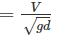Where,

V = velocity of flow through the rectangular channel

D = depth of flow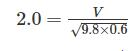V=4.85m/s

Specific Energy (E) is given by=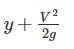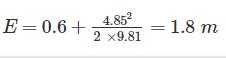QUESTION: 18

Specific gravity of water is 1.0 which is reported at a temperature of:

Solution:

Specific gravity of water is 1.0 which is reported at a temperature of 4°C. Specific gravity is a measure of density of liquid.

Some interesting facts about Specific gravity:

Specific gravity of water is actually a density of water in gram per cubic centimetre.

QUESTION: 19

To avoid vaporisation in the pipe line, the pipe line over the ridge is laid such that it is not more than _____.

Solution:

In order to avoid vaporization in the pipe line, the pipe line over the ridge is laid in such a way that it is not more than 6.4 m above the hydraulic gradient.

QUESTION: 20

Mercury is used in the barometer because:

Solution:

Mercury is used in the barometer because it is a high-density fluid which gives less height of column for high pressures. A barometer using water, for instance, would need to be 13.6 times taller than a mercury barometer to obtain the same pressure difference. This is because mercury is 13.6 times denser than water.